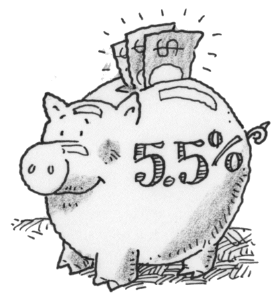### Home > APCALC > Chapter 5 > Lesson 5.3.2 > Problem5-124

5-124.

Suppose you deposit $50$ in Piggy Bank, which pays $5.5\%$ annual interest. How much money will be in your account:

1. After $6$ months if the interest is compounded twice a year?

$50\left(1+\frac{0.055}{2}\right)^1=\underline{\ \ \ \ \ \ }$

2. After $1$ year if the interest is compounded twice a year?

Refer to hint in part (a).

3. After $1$ year if the interest is compounded monthly?

$\text{investment }\left(1+\frac{\text{rate}}{\text{times compounded}}\right)^{12}=\underline{\ \ \ \ \ \ }$

4. After $2$ years if the interest is compounded monthly?

Refer to hint in part (c).

5. After $2$ years if the interest is compounded daily?

Refer to hint in part (c).